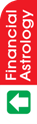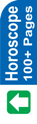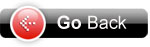Astrology Software
Indian AstrologyYOGINI DASHA Yet another popular system of dividing the entire life into major periods and periods is Yogini Dasha. This is very commonly used in hilly areas. Following are the periods as per this system. Alongwith the periods are given the number of years which show the duration of the major periods. In the third column are given the planets which affect these periods. Period Duration Lord Mangla 1 year Moon Pingla 2 years Sun Dhanya 3 years Jupiter Bhramri 4 years Mars Bhadrika 5 years Mercury Ulka 6 years Saturn Siddha 7 years Venus Sankata 8 years Rahu Total 36 years This occurs twice or even thrice in one life. To study this, just consider the position of Moon for Mangla, Sun for Pingla, and so on. If Moon is malefic in a natal horoscope, then Mangla Period would give evil results. Similarly, for others periods. Calculation of Major Periods First of all refer to the following table to find out the current period at the time of birth : ------------------------------------------------------------------------------------------------ Periods Man Pin Dha Bhr Bha Ulk Sid San ------------------------------------------------------------------------------------------------ Yrs 1 2 3 4 5 6 7 8 ------------------------------------------------------------------------------------------------ Planets Mon Sun Jup Mar Mer Sat Ven Rah ------------------------------------------------------------------------------------------------ Star 1 2 3 4 5 6 7 8 9 10 11 12 13 14 15 16 17 18 19 20 21 22 23 24 25 26 27 ------------------------------------------------------------------------------------------------ No. 1 denotes Ashwini, No. 2 Bharni, and so on. The longitude of Moon in the our sample example is : 6s 7° 35' 36" (6s 7° 35'). This means that Moon is in Libra (sign 7) and has travelled 7° 35' 36" (7° 35') in Libra. Find Nakshatra/star from the table given earlier. It shows that Swati Nakshatra is from 6° 40' to 20° 0'. Swati Nakshatra tells us, from the above table, that Pingla Period is running. Since our Moon is at 7° 35', it has to travel 12° 25' (20° 0' - 7° 35') more to complete Swati Nakshatra. Pingla period will end when Moon completes Swati Nakshatra. To find out balance of Pingla period at the time of birth, proceed as under : Formula : Distance in (°) Degrees and ( ') Minutes Multiplied by Total Period of Planet Divided by 800. Hence, (12° 25') x 2 divided by 800 Where 2 is the total period of Pingla (as given above), and 800 is the total duration of one Nakshatra in minutes, i.e. we know the duration of a Nakshatra is 13° 20' = 13 x 60 + 20 = 800). For easy calculations, convert 12° 25' into minutes, i.e. 12 x 60 + 25 = 745. Hence, 745 x 2 divided by 800 = 1 yr 10 months 11 days Add this to the date of birth to find out the period of Pingla. Date of birth 10 12 1993 Balance of Pingla 11 10 1 21 10 1995 Hence, Pingla Major period is from 10.12.1993 to 21.10.1995. Add 3 yrs for getting Dhanya Period 21.10.1998 Add 4 yrs for getting Bhramri Period 21.10.2002 Add 5 yrs for getting Bhadrika Period 21.10.2007 Add 6 yrs for getting Ulka Period 21.10.2013 Add 7 yrs for getting Siddha Period 21.10.2020 Add 8 yrs for getting Sankata Period 21.10.2028 Add 1 yr for getting Mangla Period 21.10.2029 Again, add 2 yrs for getting Pingla period, and so on. Calculation of Periods under Major Periods Now, we have to calculate periods. In each major period, there is a further sub-division of period for each planet in the same order as given above. For example, Rahu is from 10.12.1993 to 14.9.2010, as calculated above. During this major period, there will be periods of all planets, i.e. First will be the period of Pingla - Pingla ( current period of Pingla and in that first period of Pingla) followed by Pingla Dhanya, followed by Pingla-Bhramri, Pingla-Bhadrika, Pingla-Ulka, Pingla-Siddha, Pingla-Sankata, and Pingla-Mangla. We should, therefore, now calculate the exact period of Pingla-Pingla and so on. Formula : Major Period x Period ¸ 36 (Total No. of years) In the above case, first major period is of Pingla, which is for 2 years (as is clear from the above). Period is also of Pingla. Hence, (2 x 2) ¸ 36 = 1/9 years Multiply by 12 for converting into months, 1/9 x 12 = 1 3/4 months Multiply by 30 for converting into days, 3/4 x 30 = 22 1/2 days, i.e. 23 days Period of Pingla-Pingla is 1 month 23 days. This ways, all the periods can be found out.Astrowealth Astrology Software Software for Website Astrology Reports Free Services Regional Language Important Link